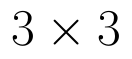Select Page

# CBSE Maths 12 Science Integrals Answers for MCQ in English

CBSE Maths 12 Science Integrals Answers for MCQ in English to enable students to get Answers in a narrative video format for the specific question.

Expert Teacher provides CBSE Integrals Maths 12 Science Answers for MCQ through Video Answers in English language. This video solution will be useful for students to understand how to write an answer in exam in order to score more marks. This teacher uses a narrative style for a question from Integrals not only to explain the proper method of answering question, but deriving right answer too.

Please find the question below and view the Answer in a narrative video format.

Question:

## Similar Questions from CBSE, 12th Science, Maths, Integrals

Question 1 : Evaluate :(View Answer Video)

Question 2 : Evaluate :(View Answer Video)

Question 3 : Write the value of :(View Answer Video)

Question 4 : Find :(View Answer Video)

Question 5 : Evaluate :(View Answer Video)

### Matrices

Question 1 : LetFind A - B. (View Answer Video)

Question 2 : Given,, find the value of y. (View Answer Video)

Question 3 : Find the value of y from the equation:(View Answer Video)

Question 4 : If, find (x-y). (View Answer Video)

Question 5 :  Find the value of y, if(View Answer Video)

### Continuity and Differentiability

Question 1 : Differentiate w.r.t.x the function. (View Answer Video)

Question 2 : Differentiate the functionwith respect to x. (View Answer Video)

Question 3 : Findfor the function. (View Answer Video)

Question 4 : Using the fact thatand the differentiation, obtain the sum formula for cosines. (View Answer Video)

Question 5 : Differentiate the functionwith respect to x. (View Answer Video)

### Determinant

Question 1 : If, then x is equal to, (View Answer Video)

Question 2 : Let A be the non- singular square matrix of order, then |adj A| is equal to, (View Answer Video)

Question 3 : Find the equation of the line joining (1, 2) and (3, 6) using determinants. (View Answer Video)

Question 4 : Iffind |A|. (View Answer Video)

Question 5 : If area of a triangle is 35 sq unit with vertices (2, -6), (5, 4) and (k, 4), then k is, (View Answer Video)